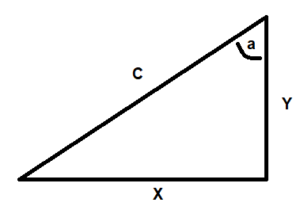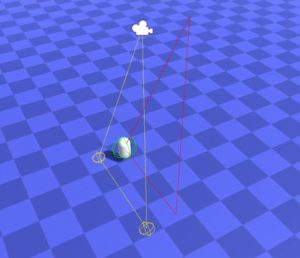# Smooth Overhead Camera for Unity

While working on a small Unity project a couple of months back I built a simple overhead camera which centers the view on the main player object and adds a nice bit of smoothing. This was made for a top-down platformer-style adventure game. I hesitate to call it a game at this point, but hey, it’s close enough for these purposes. Below you’ll find links to grab it from Github, and a break down of how it works.

This video clip shows a comparison between the unsmoothed and smoothed version.

Let’s break down how it works…

The information we have is the camera’s height (Y), and the pitch angle (a). What we need is the exact point in 3d space where the camera needs to look in order to center the player in the view. To get that we need to calculate the other two sides of the triangle.To get X, we need to take the tangent of the angle and multiply it by the height (Y), like so:

float y = transform.position.y – targetPosition.y;
float angle = 90.0f – m_cam.transform.rotation.eulerAngles.x;
float tangent = Mathf.Tan( Mathf.Deg2Rad * angle );
float x = y * tangent;

Once we have X, we can calculate the hypotenuse with the formula: a² + b² = c², which translates to
c = sqrt( (x*x) + (y*y) ). The length of the hypotenuse is used to calculate the exact center point of the screen in 3D space needed to center the player on screen, which in turn allows us to determine how far off the camera currently is from where we want it to be.

float c = Mathf.Sqrt( (x*x) + (y*y) );
Vector3 center_world_position = m_cam.transform.position + (m_cam.transform.forward * c);
Vector3 difference = (targetPosition – center_world_position);
Vector3 moveToPosition = cameraPosition + difference;
moveToPosition.y = m_cam.transform.position.y;

Since we camera height to stay the same, we set the move-to height to the current camera height. Finally, we apply interpolation to smooth out the transition.

Let’s take a quick moment to call out a great tool which Unity provides for developers. Gizmos are what allow the useful information in the scene window to let me see the current state of the camera — where it is, and where it wants to be. This was critical for getting things set up efficiently. There were math and code bugs throughout the development. Seeing these visually made a huge difference in how quickly I was able to find and fix the issues. If you only take one thing from all of this it is, use the tools to their fullest.Hope you found this useful. Let me know if you did!

Again, you can find the GitHub project here: https://github.com/bargle/Unity-SmoothOverheadCamera